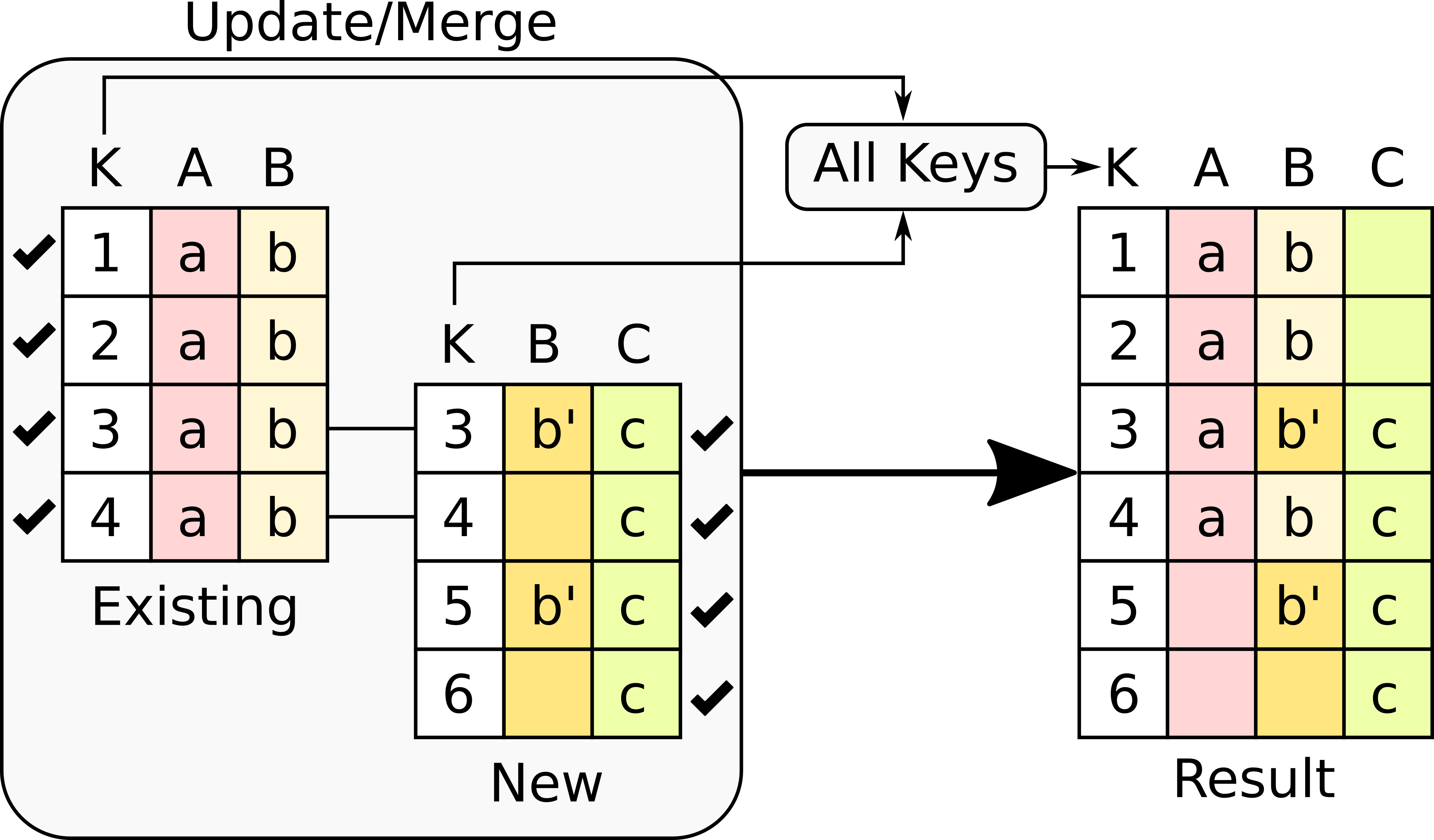# 5.23. Merging Data¶

Merging is the operation that takes place when there is a data set already stored in a table in OnTask and we combine that data with a new data set This operation is very important as a way to combine data from multiple sources. One of the most important elements for this operation to work is the presence of key columns in both the existing table and the new data. A key column uniquely identifies a row in the table. When combining two tables, a key column needs to be specified in both the existing and new data to match the rows accordingly.

Once the rows are matched using the key columns from both tables, the next step is select the rows in the result. Looking at the presence or absence of a value in both the existing table and the new table there are three possible cases:

1. There are rows with the same value in the key column in both tables.

2. There is a row with the key value in the existing table but not in the new table.

3. There is a row with the key value in the new table but not in the existing table.

Another way to look at these three cases is to think of the values in the key columns in both tables as sets. There could be elements that belong to both sets (the intersection), the existing only, or the new set only.

Considering these three types of rows, there are four possible options to combine the rows from both tables:

Use all rows from both tables

This option takes the union of all the rows in any of the tables. In other words, the resulting table will contall all rows. The following figure illustrates the effect of this option.The operation has several effects in the result.

• Column A only has values in the first four rows because there is no Column A in the new table, so it is filled out with empty values.

• Column B is present in both tables, however, the new table only contains values for rows 3 and 5. Those values are updated in the existing table, and the rest are left unchanged.

• Column C is new and only present in the new table, thus, the result has values only for that portion of the table.

Use only the rows that are present in both tables

This option takes the intersection of all the rows for which the key is present in both tables. The following figure illustrates the effect of this option.In this version of the merge operation, the number of rows is likely to be reduced because only those rows with key values in both tables are considered (the rest are dropped). More precisely, the operation has had the following effects:

• Rows with key values 1 and 2 from the existing table are removed because there is no such key in the new table.

• Rows with key values 5 and 6 in the new table are removed because there is no such key in the existing table.

• The values in Column A are left untouched because there is no such column in the new table.

• The values in Column B are updated with those in the new table, and the existing ones in table A are left untouched for those rows with no corresponding value in the new table.

• Te values in Column C are incorporated to the result.

Use only the rows in the existing table

This option takes only the key values that are present in the existing table. The following figure illustrates the effect of this option.In this version, the number of rows will be identical to those in the existing table. The rows in the new table for which there is no key value in the existing table are dropped. More precisely, the operation has had the following effects:

• Key column has the values only in the existing table.

• Column A is left untouched because it has no values in the new table.

• Column B contains the values in the existing table but updated with the non-empty values in the new table.

• Column C is present only in the new table, therefore only the values that correspond with rows in with key in the existing table are kept.

Use only the rows in the new table

This option takes only the key values that are present in the new table. The following figure illustrates the effect of this option.In this version, the number of rows in the result is identical to those in the new table. The rows in the existing table for which there is no key value in the new table are dropped. More precisely, the operation has had the following effects:

• Key column has the values only in the new table.

• Column A only contains the values in the existing table because the rest had not value in the new table.

• Column B has the values taken from the new table and the existing one for those rows for which there is no value in the new table.

• Column C contains the values in the new table untouched.

Why so many options? These options are useful for scenarios that may match the effects described above. In some cases the new tables bring additional rows that need to be added to the existing ones, or replace the existing ones. In other scenarios the existing table takes precedence and the new rows in the new table are dropped. In other sets of scenarios you would like to keep those rows that are in both data tables.